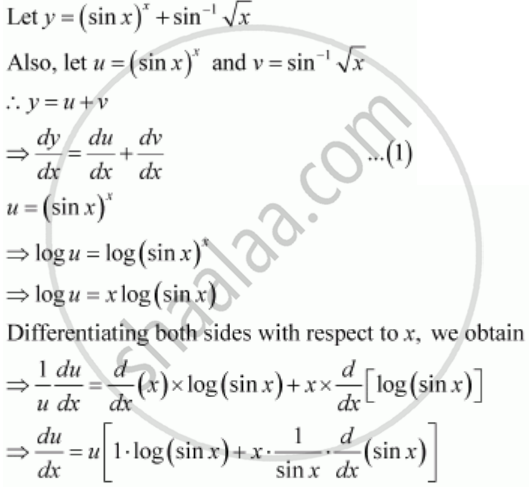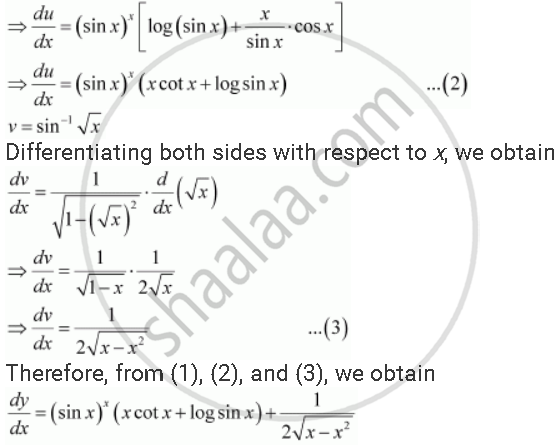# Differentiate the Function with Respect To X.(Sin X)^X + Sin^(-1) Sqrtx - Mathematics

Differentiate the function with respect to x.

(sin x)^x + sin^(-1) sqrtx

Differentiate the function (sin x)^x + sin^(-1) sqrtx with respect to x

#### SolutionConcept: Logarithmic Differentiation
Is there an error in this question or solution?
Chapter 5: Continuity and Differentiability - Exercise 5.5 [Page 178]

#### APPEARS IN

NCERT Class 12 Maths
Chapter 5 Continuity and Differentiability
Exercise 5.5 | Q 8 | Page 178
Share